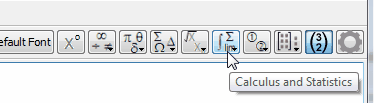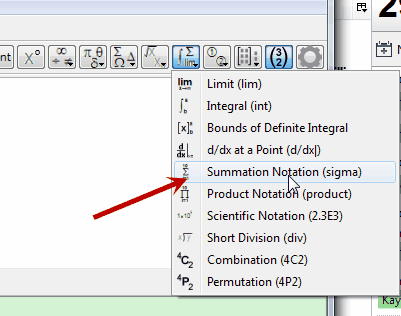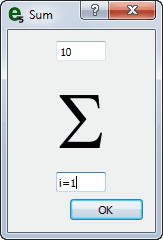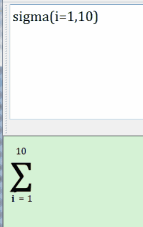﻿ Toolbar Equation Entry

# Toolbar Equation Entry

Top  Previous  Next
 FX Equation is designed to be used entirely from the keyboard but that relies on users remembering the codes to produce symbols and equation components.  Toolbar Equation Entry allows you to construct equations without needing to remember all the codes.   Toolbar Equation Entry also makes creation of some output, in particular piecewise defined functions and matrices, easier than just using the keyboard.   To use the Toolbar Equation Entry System:   1.        Move to the toolbar2.        Click the button and a list of options will appear.  Move to the desired tool and click again.3.        Sometimes FX Equation will just type the required command automatically.  Other times, FX Equation will give you the opportunity to fill in some blanks.4.        Push OK and FX Equation will build the required equation for you.  Don't forget to look at the command FX Equation used so you can type your own next time (if you wish).5.        The degrees button has no options, it will just add the degrees sign to your equation.